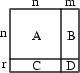# Discrete State-Space

Implement discrete state-space system

•Libraries:

## Description

### Block Behavior for Non-Empty Matrices

The Discrete State-Space block implements the system described by

`$\begin{array}{c}x\left(n+1\right)=Ax\left(n\right)+Bu\left(n\right)\\ y\left(n\right)=Cx\left(n\right)+Du\left(n\right),\end{array}$`

where u is the input, x is the state, and y is the output. The matrix coefficients must have these characteristics, as illustrated in the following diagram:

• A must be an n-by-n matrix, where n is the number of states.

• B must be an n-by-m matrix, where m is the number of inputs.

• C must be an r-by-n matrix, where r is the number of outputs.

• D must be an r-by-m matrix.The block accepts one input and generates one output. The width of the input vector is the number of columns in the B and D matrices. The width of the output vector is the number of rows in the C and D matrices. To define the initial state vector, use the Initial conditions parameter.

To specify a vector or matrix of zeros for A, B, C, D, or Initial conditions, use the `zeros` function.

### Block Behavior for Empty Matrices

When the matrices A, B, and C are empty (for example, `[]`), the functionality of the block becomes `y(n) = Du(n)`. If the Initial conditions vector is also empty, the block uses an initial state vector of zeros.

## Ports

### Input

expand all

Input vector, where the width equals the number of columns in the B and D matrices. For more information, see Description.

Tip

For integer and fixed-point input signals, use the Fixed-Point State-Space block.

Data Types: `single` | `double`

### Output

expand all

Output vector, with width equal to the number of rows in the C and D matrices. For more information, see Description.

Data Types: `single` | `double`

## Parameters

expand all

### Main

Specify the matrix coefficient `A`, as a real-valued n-by-n matrix, where n is the number of states. For more information on the matrix coefficients, see Description.

#### Programmatic Use

 Block Parameter: `A` Type: character vector Values: scalar | vector | matrix Default: `'1'`

Specify the matrix coefficient `B`, as a real-valued n-by-m matrix, where n is the number of states, and m is the number of inputs. For more information on the matrix coefficients, see Description.

#### Programmatic Use

 Block Parameter: `B` Type: character vector Values: scalar | vector | matrix Default: `'1'`

Specify the matrix coefficient C, as a real-valued r-by-n matrix, where r is the number of outputs, and n is the number of states. For more information on the matrix coefficients, see Description.

#### Programmatic Use

 Block Parameter: `C` Type: character vector Values: scalar | vector | matrix Default: `'1'`

Specify the matrix coefficient D, as a real-valued r-by-m matrix, where r is the number of outputs, and m is the number of inputs. For more information on the matrix coefficients, see Description.

#### Programmatic Use

 Block Parameter: `D` Type: character vector Values: scalar | vector | matrix Default: `'1'`

Specify the initial state vector as a scalar or vector. The initial state vector cannot include `inf` or `NaN` values.

#### Programmatic Use

 Block Parameter: `InitialCondition` Type: character vector Values: scalar | vector Default: `'0'`

Specify the time interval between samples. See Specify Sample Time.

#### Programmatic Use

 Block Parameter: `SampleTime` Type: character vector Values: scalar | vector Default: `'-1'`

### State Attributes

Use this parameter to assign a unique name to the block state. The default is `' '`. When this field is blank, no name is assigned. When using this parameter, remember these considerations:

• A valid identifier starts with an alphabetic or underscore character, followed by alphanumeric or underscore characters.

• The state name applies only to the selected block.

This parameter enables State name must resolve to Simulink signal object when you click Apply.

#### Programmatic Use

 Block Parameter: `StateName` Type: character vector Values: unique name Default: `''`

Select this check box to require that the state name resolves to a Simulink® signal object.

#### Dependencies

To enable this parameter, specify a value for State name. This parameter appears only if you set the model configuration parameter Signal resolution to a value other than `None`.

#### Programmatic Use

 Block Parameter: `StateMustResolveToSignalObject` Type: character vector Values: `'off' | 'on'` Default: `'off'`

## Block Characteristics

 Data Types `double` | `single` Direct Feedthrough `yes` Multidimensional Signals `no` Variable-Size Signals `no` Zero-Crossing Detection `no`

## Version History

Introduced before R2006a